# Year 11 ATAR Course Textbook - Mathematics Methods

\$75.00
Buy 1 to 4: \$75.00 each
Buy 5 to 9: \$71.25 each
Buy 10 or more: \$67.50 each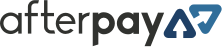Available on orders \$80 to \$1000Available on orders \$80 to \$1000

Year 11 ATAR Course Textbook - Mathematics Methods is a comprehensive textbook for the year 11 Mathematics Methods Units 1 & 2 course.

Availability: Out of Stock Usually Dispatched In: 3 - 7 Business Days ISBN: 9781740981743 Publisher: Academic Task Force

Year 11 ATAR Course Textbook - Mathematics Methods is a comprehensive textbook for the year 11 Mathematics Methods Units 1 & 2 course.

#### Contents

• 1 Lines
• 1.1 Independent & Dependent Variables
• 1.2 Equation of Lines
• 1.2.1 Gradient-Intercept form: y = mx + c
• 1.2.2 General form: ax + by = c
• 1.2.3 Gradient of a Line
• 1.2.4 Equation of a line passing through a fixed point with a given gradient
• 1.3 Parallel and Perpendicular Lines
• 1.4 Mid-Points and Distances
• 1.5 Linear Relationships
• 1.5.1 Direct Proportion
• 1.5.2 Applications involving linear relationships
• 2.1.2 Features of quadratic curves
• 2.2 Completion of Squares
• 2.3.1 Algebraic techniques for solving quadratic equations
• 2.3.2 Existence of solutions
• 2.3.3 Working with quadratic inequalities
• 2.4 Applications Using Quadratic Models
• 3 Cubics
• 3.1 Cubics
• 3.2 Features of Cubics (cubic curves)
• 3.2.1 Cubics with one root
• 3.2.2 Cubics with two roots
• 3.2.3 Cubics with three roots
• 3.3 Solving cubic equations
• 4 Rectangular Hyperbolas
• 4.1 Inverse Proportion
• 4.2 Rectangular Hyperbolas (hyperbolae)
• 5 Functions & Relations
• 5.1 Definition of a Relation
• 5.2 Definition of a Function
• 5.3 Polynomials
• 5.4 Domain and Range
• 5.4.1 Square Root Functions
• 5.4.2 Exponential Functions
• 5.5 Graphs of some relations
• 5.5.1 Circles
• 5.5.2 The relation y2 = kx
• 5.6 Transformations on functions
• 5.7 Compound Transformations
• 6 Right Triangle Trigonometry
• 6.1 Trigonometric Ratios
• 6.1.2 Exact Ratios
• 7 Trigonometric Ratios of non-acute angles
• 7.1 Review of Trigonometric Ratios of acute angles
• 7.3 Reference Angles
• 7.4 Circular Functions
• 7.5 Trigonometric ratios of non-acute angles
• 7.5.1 Negative angles
• 7.7 Inverse Trigonometric Functions
• 7.7.1 Principal Values
• 8 Non-Right Triangle Trigonometry
• 8.1 The Sine Rule
• 8.2 Cosine Rule
• 8.3 Area of a non-right triangle
• 9 Arc, Sectors and Segments
• 9.1 Arcs, Sectors and Segments
• 10 Trigonometric Equations
• 10.1 Basic Trigonometric Equations
• 10.2 Gradient of a Line
• 10.3 Use of CAS Calculators
• 10.3.1 Extension: General Solutions
• 10.3.2 Using the “Conditional Solve” CAS command
• 10.4 Basic Trigonometric Ratios within polynomials
• 11 Trigonometric Graphs
• 11.1 Graphs of basic trigonometric functions
• 11.2 Graphs of Trigonometric functions of the form y = a f (x + c) + d
• 12 Trigonometric Identities
• 12.1 Compound Angle Formulae
• 13 Sets
• 13.1 Set Notation
• 14 Combinations
• 14.1 The Combinatorial Notation
• 14.2 Combinations or Selections
• 14.2.1 The Multiplication and Addition Rule
• 14.3 Binomial Expansions
• 15 Probability
• 15.1 Sample Spaces
• 15.2 Theoretical (A Priori) Probability
• 15.3 Empirical (A Posteriori) Probability
• 15.4 Combining Events & Conditional Probability I
• 15.5 Conditional Probability II
• 15.6 Rules of Probability
• 16 Indices
• 16.1 Review of the Laws of Indices
• 16.2 Review of Negative Indices
• 16.3 Review of Fractional Indices
• 16.4 Review: Factorisation
• 16.5 Review: Scientific Notation
• 17 Exponential Functions
• 17.1 Exponential Functions
• 17.2 Exponential Expressions and Equations
• 18 Sequences & Series
• 18.1 Recursive Rules
• 18.2 Arithmetic Sequences
• 18.2.1 Sum of an arithmetic sequence
• 18.3 Geometric Sequences
• 18.3.1 Types of Geometric Sequences
• 18.3.2 Geometric Sum
• 18.3.3 Sum to Infinity
• 18.4 Applications
• 19 Differentiation
• 19.1 Tangents and Chords
• 19.2 Gradient of a Curve at a Point
• 19.2.1 Analytical Method for determining Gradient of a Curve at a Point
• 19.3 Differentiation of Polynomials
• 20.1 Equation of tangent to a Curve
• 20.2 Graphs of Gradient Functions and Stationary Points
• 20.3 Stationary Points
• 20.4 Curve Sketching
• 21 Rates of Change
• 21.1 Instantaneous Rate of Change
• 21.1.1 The Leibniz Notation
• 21.2 Optimisation
• 21.2.1 Global Points and Turning Points
• 21.3 More Optimisation
• 22 Anti-Differentiation
• 22.1 Anti-Differentiation of Polynomials
• 22.1.1 Rules for Anti-Differentiation
• 22.2 Gradient Function and Integration
• 23 Rectilinear Motion0
• 23.1 Rectilinear Motion and Differentiation
• 23.2 Rectilinear Motion and Integration
• 23.3 Acceleration
• Index

## Also Available

#### Year 11 ATAR Course Study Guide - Mathematics Methods

The purpose of this text is to assist Year 11 students with their preparation for tests and examinations in the Mathematical Methods course for Western Australia.

#### Year 11 ATAR Course Revision Series - Mathematics Methods

Year 11 ATAR Course Revision Series - Mathematics Methods provides a comprehensive set of revision/review questions for the year 11 Mathematics Methods Units 1 & 2 course.

 ISBN 9781740981743 Publisher Academic Task Force Product Type Student Books, Year Level Year 11, Author(s) Dr O. T. Lee

#### Be The First To Review This Product!

Help other Teacher Superstore users shop smarter by writing reviews for products you have purchased.

### We Also Recommend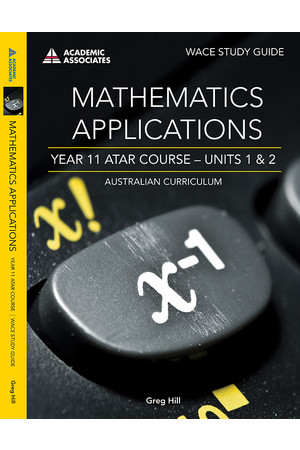### Year 11 ATAR Course Study Guide - Mathematics Applications

\$45.00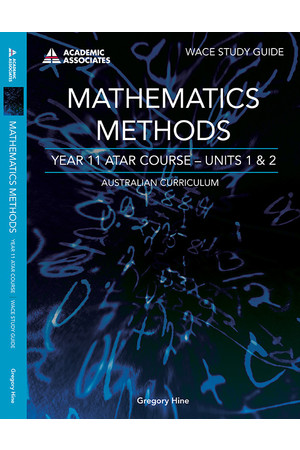### Year 11 ATAR Course Study Guide - Mathematics Methods

\$45.00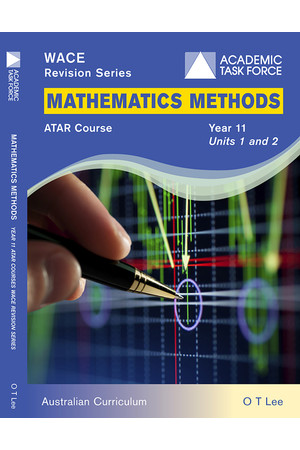\$49.00

### Others Also Bought### Year 11 ATAR Course Study Guide - Mathematics Methods

\$45.00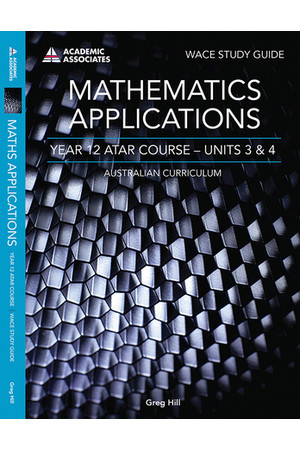### Year 12 ATAR Course Study Guide - Mathematics Applications

\$49.00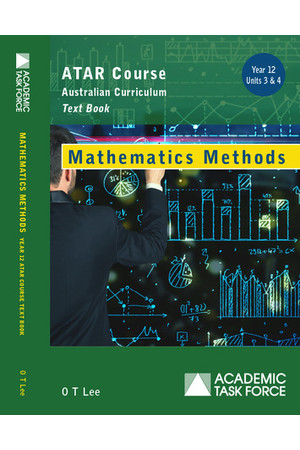### Year 12 ATAR Course Textbook - Mathematics Methods

\$80.00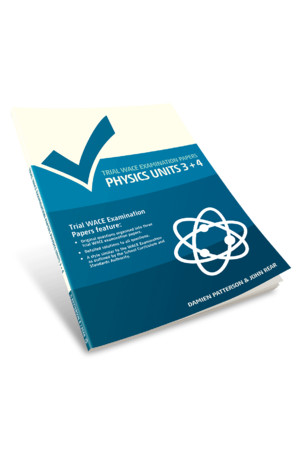### Trial WACE Examination Papers – Physics: Units 3 + 4

\$39.95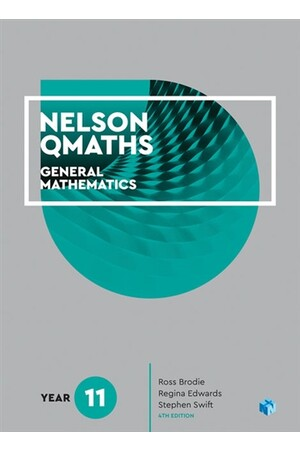### Nelson QMaths: Mathematics General - Year 11 (Student Book with 4 Access Codes)

\$79.95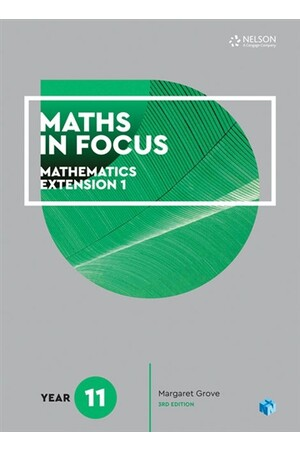### Maths in Focus: Mathematics Extension 1 - Year 11 (Student Book with 1 Access Code for 26 Months)

\$79.95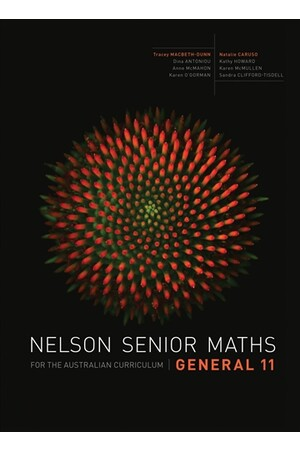### Nelson Senior Maths General for the Australian Curriculum - Year 11: Student Book

\$88.95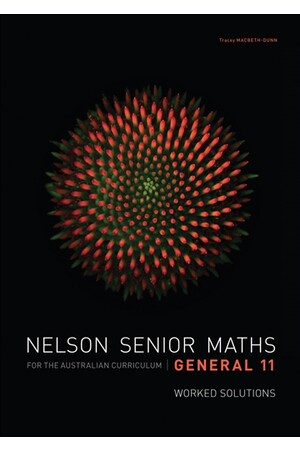\$51.95

### More From This Category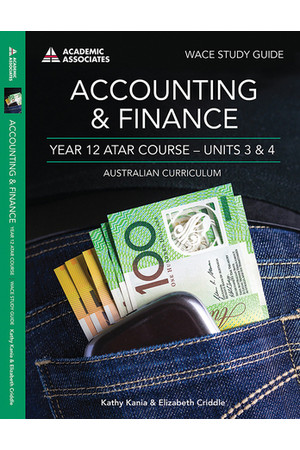### Year 12 ATAR Course Study Guide - Accounting & Finance

\$49.00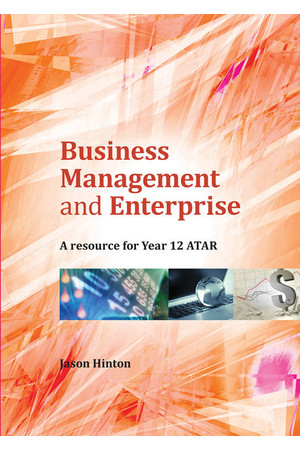### Business Management and Enterprise: A Resource for Year 12 ATAR

\$64.95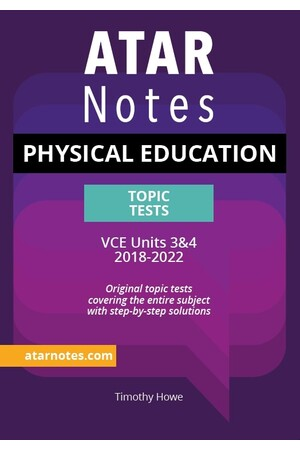### ATAR Notes VCE Physical Education 3 & 4 Topic Tests

\$37.00### ATAR Notes Text Guide: In Cold Blood by Truman Capote

\$17.00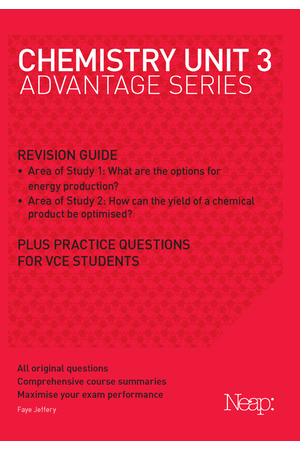### Neap Advantage Series: VCE Chemistry Unit 3 (2017 Ed)

\$24.95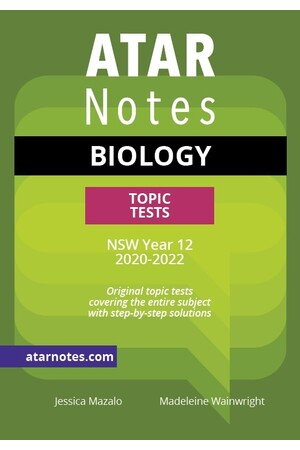### ATAR Notes Year 12 Biology Topic Tests - NSW

\$37.00### ATAR Notes HSC Survival Guide

\$20.00\$17.00